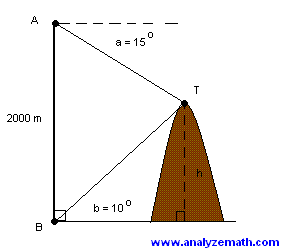# Solve Trigonometry Problems

 Trigonometry problems with detailed solution are presented. Problem 1: A person 100 meters from the base of a tree, observes that the angle between the ground and the top of the tree is 18 degrees. Estimate the height h of the tree to the nearest tenth of a meter.Solution to Problem 1: Use the tangent tan(18o) = h / 100 Solve for h to obtain h = 100 tan(18o) = 32.5 meters. Problem 2: The angle of elevation of a hot air balloon, climbing vertically, changes from 25 degrees at 10:00 am to 60 degrees at 10:02 am. The point of observation of the angle of elevation is situated 300 meters away from the take off point. What is the upward speed, assumed constant, of the balloon? Give the answer in meters per second and round to two decimal places.Solution to Problem 2: Use the tangent to write tan(25o) = h1 / 300 and tan(60o) = (h1 + h2) / 300 Solve for h1 and h2 h1 = 300 tan(tan(25o)) and h1 + h2 = 300 tan(60o) Use the last two equations to find h2 h2 = 300 [ tan(60o) - tan(25o) ] If it takes the balloon 2 minutes (10:00 to 10:02) to climb h2, the the upward speed S is given by S = h2 / 2 minutes = 300 [ tan(60o) - tan(25o) ] / (2 * 60) = 3.16 m/sec Problem 3: Point P has initially coordinates (x,y). It is then rotated by angle a about the origin to point P' (the distance r from the origin is conserved). What are the new coordinates (x',y') of point P'.Solution to Problem 3: Express x , y , x' and y' using angles b and a + b as follows x = r cos b y = r sin b x' = r cos(a + b) y' = r sin(a + b) and Expand x' and y'. x' = r cos(a + b) = r cos a cos b - r sin a sin b y' = r sin(a + b) = r sin a cos b + r cos a sin b We now use x = r cos b and y = r sin b in the above expressions to obtain x' = x cos a - y sin a y' = x sin a + y cos a The above relationships between x, y , x' and y' may be written in matrix form as followsProblem 4:An airplane is approaching point A along a straight line and at a constant altitude h. At 10:00 am, the angle of elevation of the airplane is 20o and at 10:01 it is 60o. What is the altitude h of the airplane if the speed of the airplane is constant and equal to 600 miles/hour? (round answer to 2 decimal places).Solution to Problem 4: We first calculate distance d using the time and speed (1 minute = 1/60 hour) d = 600 * (1 / 60) = 10 miles We next express the tangent of the given angles of elevation as follows tan(20o) = h / (d + x) and tan(60o) = h / x Eliminate x in the two equations above to find a relationship between h and d h = d / [ 1 / tan(20o) - 1 / tan(60o) ] = 4.6 miles (rounded to 2 decimal places) Problem 5: When the top T of a mountain is viewed from point A, 2000 m from ground, the angle of depression a is equal to 15o and when it is viewed from point B on the ground the angle of elevation b is equal to 10o. If points A and B are on the same vertical line, find the height h of the mountain. (round answer to one decimal place).Solution to Problem 5: Let h be the height of the mountain as shown in the figure below. Use the right triangles MTB and MTA to writetan(10o) = h / d tan(15o) = (2000 - h) / d Solve for d the last 2 equations as follows d = h / tan(10o) and d = (2000 - h) / tan(15o) and eliminate d as follows h / tan(10o) = (2000 - h) / tan(15o) Solve the above for h h = 2000 tan(10o) / [ tan(15o) + tan(10o)] = 793.8 m (rounded to 1 decimal place) More Trigonometry Problems